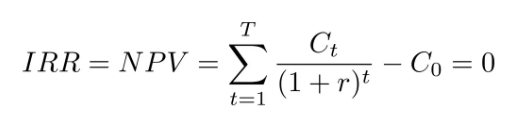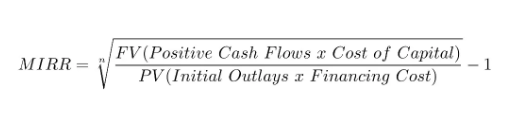# Difference Between IRR and MIRR## What is IRR?

Internal rate of return or IRR is a measure in capital budgeting parlance, which is used to estimate the profit that can be obtained from the investments.

Internal rate of return is a type of discount rate that is used to make the net present value of all the cash flows from any project equal to zero.

In simple words, it can be referred to as the compounded annual rate of return that can be earned on an investment or a project.## What is MIRR?

The modified internal rate of return (MIRR) presumes that constructive cash flows are reinvested to the company’s cost of capital and that the inceptive outlays are funded at the company’s financing cost. It is a development over IRR and changes many things like: it deletes the different IRRs, checks the reinvestment price issues and initiates outcome, which is in a link with the present value method.Here are some points of difference between IRR and MIRR:

 IRR MIRR Definition IRR is the discount amount for investment that corresponds between the initial capital outlay and the present value of predicted cash flows. MIRR is the price in the investment plan that equalises the latest value of the cash inflow to the first cash outflow. What does it mean? It is a discount rate at which the NPV of all the cash flows from a project becomes zero. It is a modified form of IRR in which the NPV of the inflows (cash flows) is equal to the outflows (investments). Basis of assumption It assumes that the positive cash flows from a project are reinvested on the same rate of return as that of investments. It assumes that positive cash flows are reinvested based on the cost of the capital of the firm. Precision IRR is comparatively less precise in calculating the rate of return. MIRR is much more precise than IRR.

This concept was about the difference between IRR and MIRR. Stay tuned for questions papers, sample papers, syllabus, and relevant notifications on our website.